# Digital Electronics MCQ Level - 2

## 10 Questions MCQ Test Solid State Physics, Devices & Electronics | Digital Electronics MCQ Level - 2

Description
Attempt Digital Electronics MCQ Level - 2 | 10 questions in 45 minutes | Mock test for IIT JAM preparation | Free important questions MCQ to study Solid State Physics, Devices & Electronics for IIT JAM Exam | Download free PDF with solutions
QUESTION: 1

### A logic circuit with an output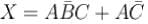consists of :

Solution: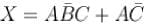can be implemented through the following diagram,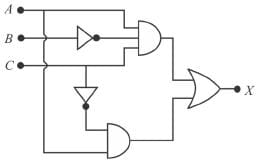Which requires 2 AND gate, 1 OR gate and two inverters The correct answer is: two AND gates, one OR gate and two inverter

QUESTION: 2

### In the given digital logic circuit, A and B form the inputs. The output Y is: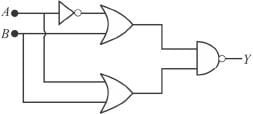Solution: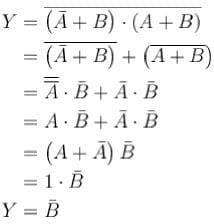The correct answer is: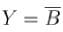QUESTION: 3

### How many entries will be in the truth table of a 4-input NAND gate?

Solution:

A NAND gate is a universal logic gate that performs the negation (NOT) of an AND logic operations in digital circuits.
As we know,
Y = 2
Y number of Entries in the truth table Where, n = number of inputs.

QUESTION: 4

A half-adder is a digital circuit with :

Solution: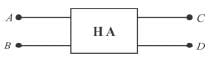The algebraic expression of half-adder are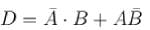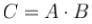The correct answer is: two inputs and two outputs

QUESTION: 5

In the given figure, circuit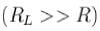performs the operation of :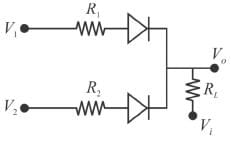Solution:

If either V1 is positive or V2 is positive, V0 be positive, otherwise we get a low output for both V1 and V2 negative. Hence it acts as an OR gate.
The correct answer is: OR gate for a negative logic system

QUESTION: 6

The circuit shown in the figure below functions as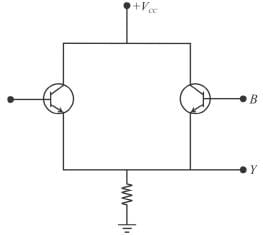Solution:

When A is high and B is low i.e. A = 1, B = 0 transistor A conducts and Y becomes high, i.e. Y = 1. If A = 0 and B = 1; transistor B conducts Y = 1, if A = 1 and B = 1; both transistors conduct. If A = B = 0. None of the transistors conducts hence Y= 0. The true table is shown below.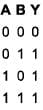The correct answer is: OR gate

QUESTION: 7

The diagram of a logic circuit is given below. The output F is best represented as.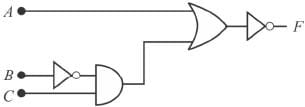Solution:

From the figure,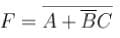The correct answer is: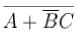QUESTION: 8

Which one of the following gives the output Ffor the logic diagram shown below?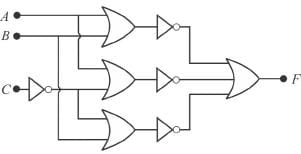Solution: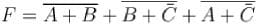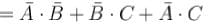The correct answer is: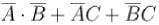QUESTION: 9

The simplest logic gate circuit corresponding to Boolean expression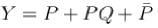is:

Solution:

Y=P+PQ+P=P(1+Q)+P=P+P=1

The output matches with

Y=P+P+Q=1+Q=1

Hence, logic for Y=P+PQ+P.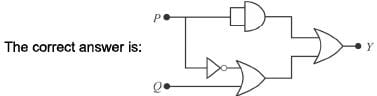QUESTION: 10

The number of bits that a digital computer can process in parallel at a time is called :

Solution:

Word length.
The correct answer is: word lengthUse Code STAYHOME200 and get INR 200 additional OFF Use Coupon Code